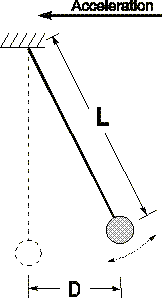# Pendulum accelerometer problem

• skiracer88_00

## Homework Statement

A pendulum has a length L = 1.04 m, with mass m=0.43 kg at the end. It hangs straight down in a jet plane about to take off as shown by the dotted line in the figure. The jet then accelerates uniformly, and while the plane is accelerating, the equilibrium position of the pendulum shifts to the position shown by the solid line, with D = 0.380 m. Calculate the magnitude of the plane's acceleration.F=ma

## The Attempt at a Solution

I really don't know where to start with this problem.. I drew a free body diagram for the pendulum weight and didnt know what to do next

Thanks for anyone who can help :)

First identify all the forces acting on the mass. (If you've drawn your free body diagram, you've got that step done.) Now apply Newton's 2nd law to both vertical and horizontal components.